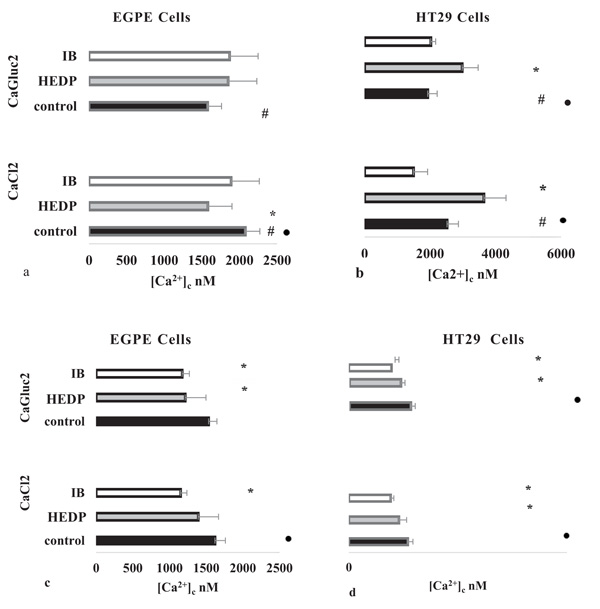Fig. (3) Ionic Calcium Concentration in Parasite’ EGPE and Mammalian’ HT29 cells. The [Ca2+]c was measured on whole cell in different bath medium conditions: (a) and (b) hyperpolarized:149 mM [Na+]o and 2.22 mM [K+]o and (c) and (d) depolarized: 26 mM [Na+]o and 125.22 [K+]o. Cells were challenged with calcium salts 1.50 mM CaCl2 or CaGluc2 (control). In samples 30 µM etidronate (HEDP) or ibandronate (IB) were added before calcium salts. Measure were performed on cells in suspension labelled with Fluo-4,AM and seeded in black 96 wells, as described. Fluorescence was measured in Ex/ Em wavelengths, 495-516 nm, 25° C. The [Ca2+]c was calculated as it was described. Results were evaluated by “student’s t” test. (#) p< 0.05 in cells EGPE or HT29 cells, comparing the effect on ionic calcium triggered by the different calcium salts without BF, in the same bath conditions. Hyperpolarized cell condition, without BF, control (a) EGPE cells with CaCl2 n=46 and CaGluc2, n=43; (b) HT29 cells with CaCl2 n=22 and CaGluc2 n=37. Depolarized cell condition, without BF, control (c) EGPE cells with CaCl2 n=4 and CaGluc2 n=18; (d) HT29 cells with CaCl2 n=15 and CaGluc2 n=19. (*) p< 0.05 in cells EGPE or HT29 between controls and samples: cells defied with different BF and calcium salts. In hyperpolarization, [Na+]o and HEDP (a) EGPE cells: CaCl2; n=11and CaGluc2; n=11; (b) HT29 cells: CaCl2; n=8 and CaGluc2 n=10. In depolarization, [K+]o and HEDP (c) EGPE cells: CaCl2 n=11 and CaGluc2 n=3; (d) HT29 cells: CaCl2 n=9 and CaGluc2 n=7. In hyperpolarization and IB (a) EGPE cells: CaCl2 n=10 and CaGluc2 n=10; (b) HT29 cells: CaCl2 n=8 and CaGluc2 n=7. In depolarized cells and IB (c) EGPE cells: CaCl2 n=9 and CaGluc2 n=10; (d) HT29 cells: CaCl2 n=9 and CaGluc2 n=10. Effect of the polarized state on [Ca2+]c (●) p<0.05 between the values obtained in the same cell defied with the same calcium salt changing bath’ ionic composition: [Na+]o and [K+]o, in EGPE (a and c) or HT29, (b and d).